# Chemical Reactions Types of Reactions There are five

• Slides: 29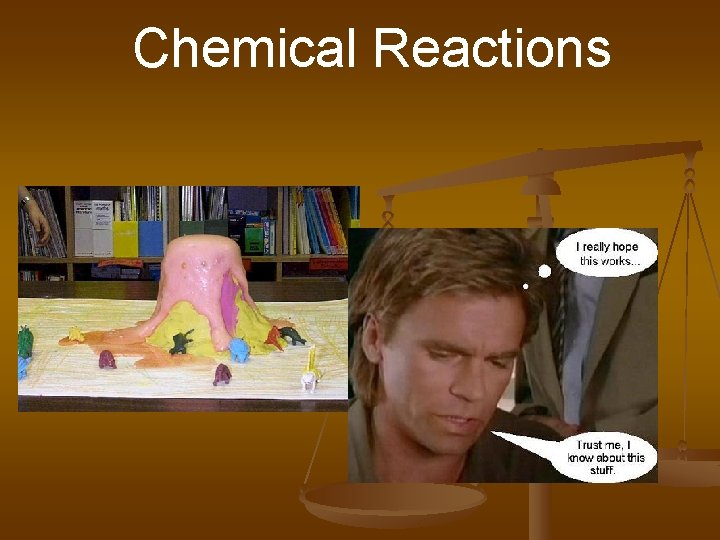Chemical Reactions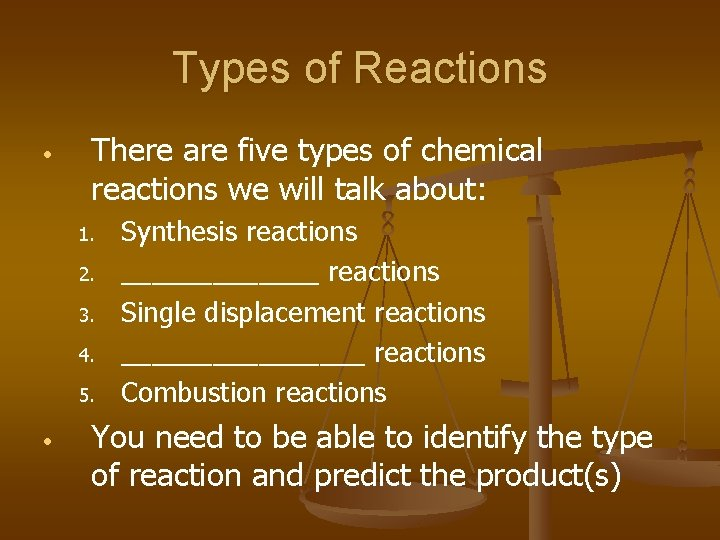Types of Reactions • There are five types of chemical reactions we will talk about: 1. 2. 3. 4. 5. • Synthesis reactions _______ reactions Single displacement reactions ________ reactions Combustion reactions You need to be able to identify the type of reaction and predict the product(s)Steps to Writing Reactions • Some steps for doing reactions 1. 2. 3. Identify the type of reaction Predict the product(s) using the type of reaction as a model Balance it Don’t forget about the diatomic elements! (Br. INCl. HOF) For example, Oxygen is O 2 as an element. In a compound, it can’t be a diatomic element because it’s not an element anymore, it’s a compound!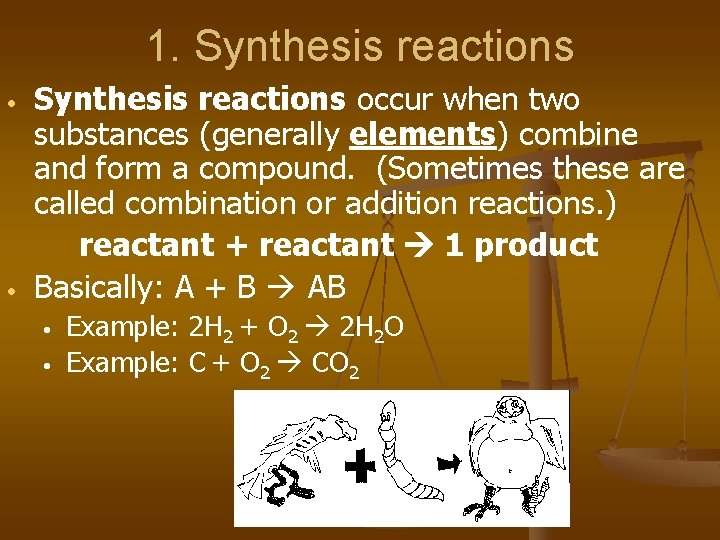1. Synthesis reactions • • Synthesis reactions occur when two substances (generally elements) combine and form a compound. (Sometimes these are called combination or addition reactions. ) reactant + reactant 1 product Basically: A + B AB • • Example: 2 H 2 + O 2 2 H 2 O Example: C + O 2 CO 2Synthesis Reactions • Here is another example of a synthesis reaction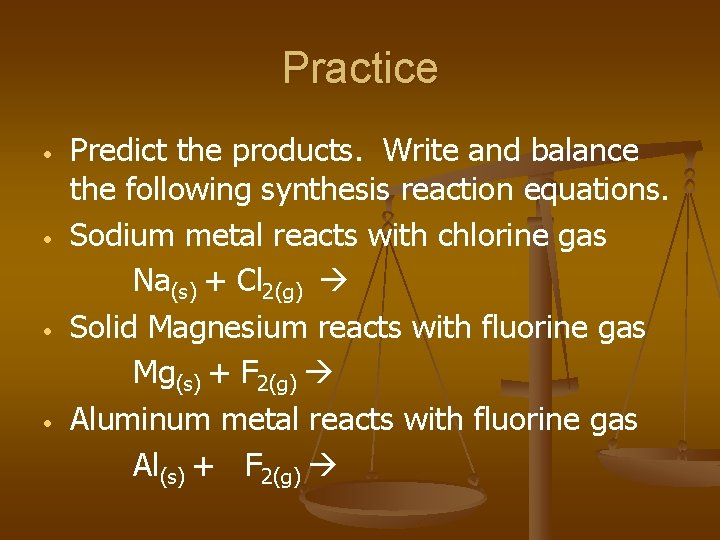Practice • • Predict the products. Write and balance the following synthesis reaction equations. Sodium metal reacts with chlorine gas Na(s) + Cl 2(g) Solid Magnesium reacts with fluorine gas Mg(s) + F 2(g) Aluminum metal reacts with fluorine gas Al(s) + F 2(g)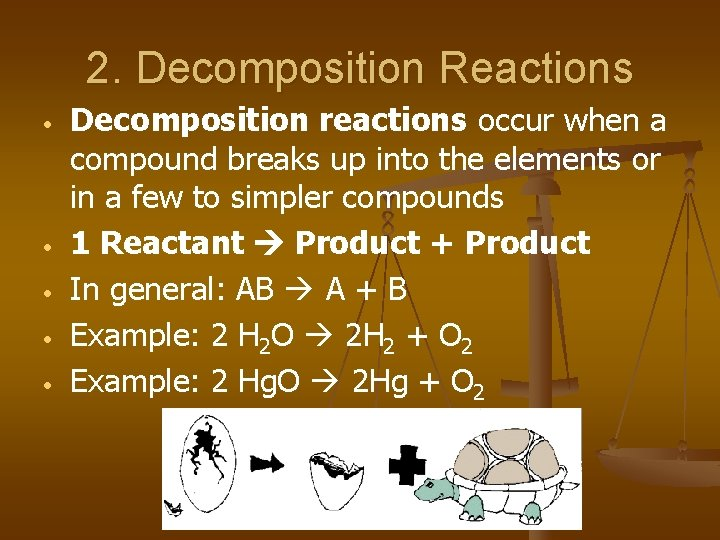2. Decomposition Reactions • • • Decomposition reactions occur when a compound breaks up into the elements or in a few to simpler compounds 1 Reactant Product + Product In general: AB A + B Example: 2 H 2 O 2 H 2 + O 2 Example: 2 Hg. O 2 Hg + O 2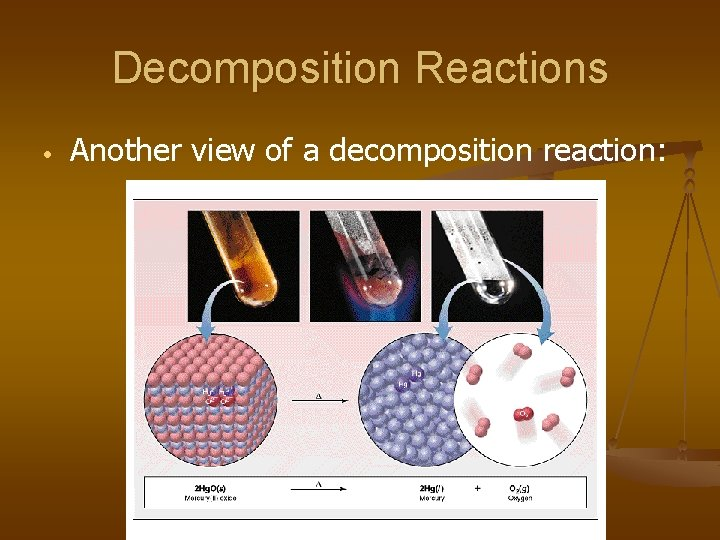Decomposition Reactions • Another view of a decomposition reaction: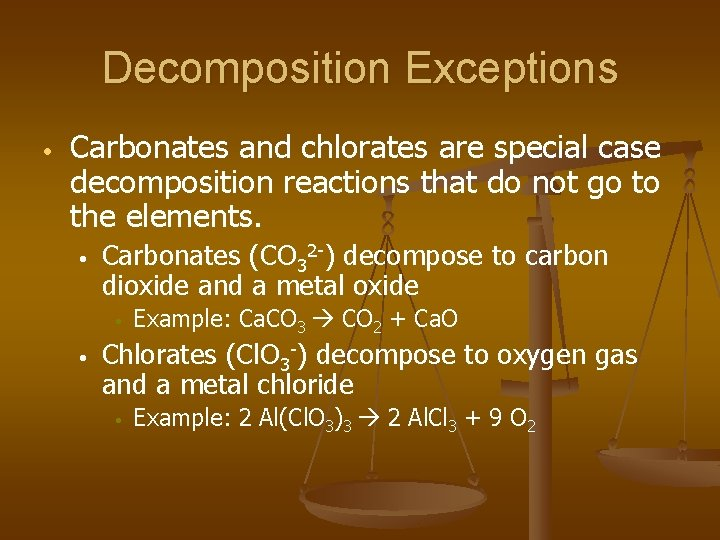Decomposition Exceptions • Carbonates and chlorates are special case decomposition reactions that do not go to the elements. • Carbonates (CO 32 -) decompose to carbon dioxide and a metal oxide • • Example: Ca. CO 3 CO 2 + Ca. O Chlorates (Cl. O 3 -) decompose to oxygen gas and a metal chloride • Example: 2 Al(Cl. O 3)3 2 Al. Cl 3 + 9 O 2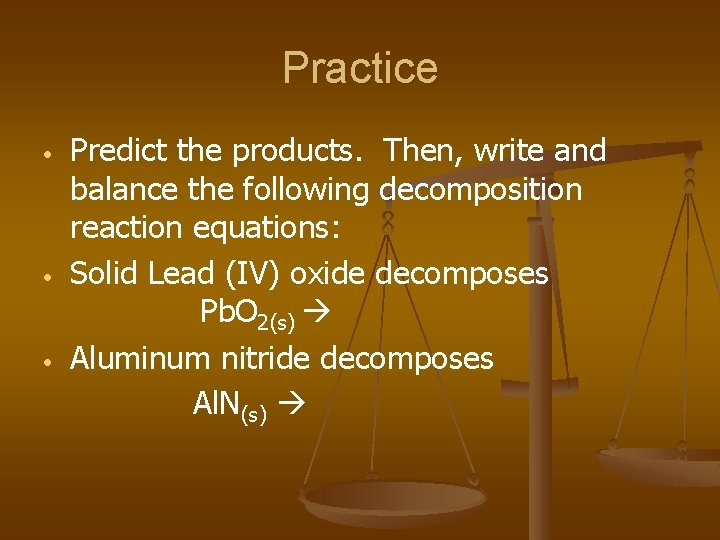Practice • • • Predict the products. Then, write and balance the following decomposition reaction equations: Solid Lead (IV) oxide decomposes Pb. O 2(s) Aluminum nitride decomposes Al. N(s)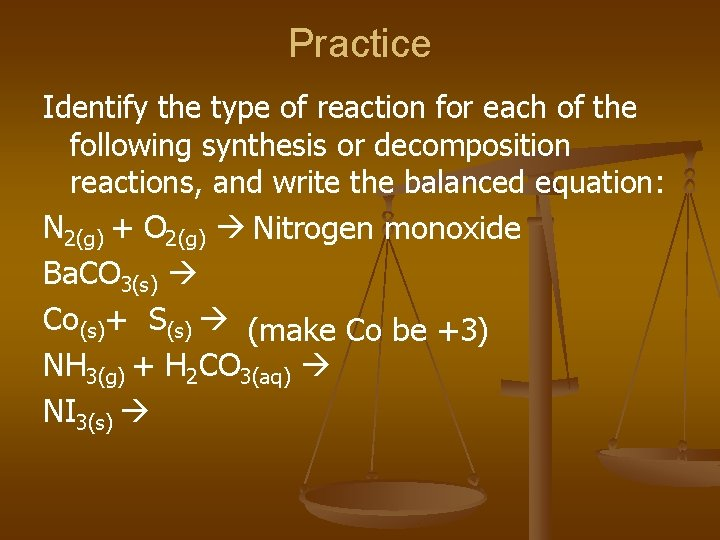Practice Identify the type of reaction for each of the following synthesis or decomposition reactions, and write the balanced equation: N 2(g) + O 2(g) Nitrogen monoxide Ba. CO 3(s) Co(s)+ S(s) (make Co be +3) NH 3(g) + H 2 CO 3(aq) NI 3(s)3. Single Replacement Reactions • • • Single Replacement Reactions occur when one element replaces another in a compound. A metal can replace a metal (+) OR a nonmetal can replace a nonmetal (-). element + compound A + BC AC + B (if A is a metal) OR A + BC BA + C (if A is a nonmetal) (remember the cation always goes first!) When H 2 O splits into ions, it splits into H+ and OH- (not H+ and O-2 !!)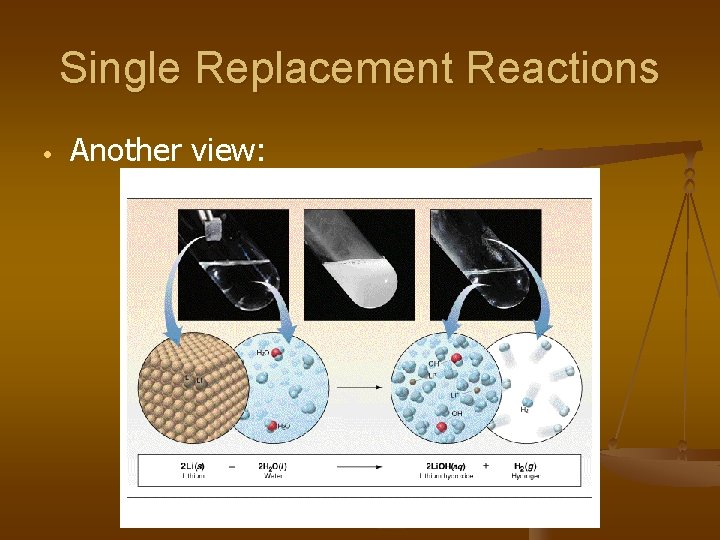Single Replacement Reactions • Another view: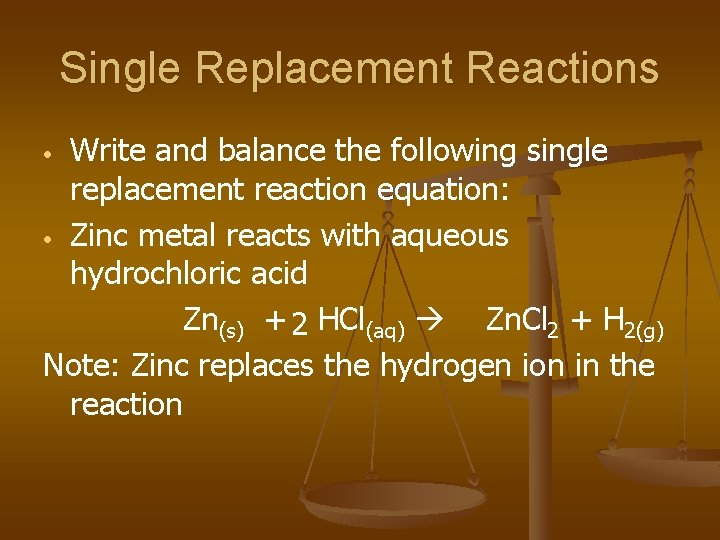Single Replacement Reactions Write and balance the following single replacement reaction equation: • Zinc metal reacts with aqueous hydrochloric acid Zn(s) + 2 HCl(aq) Zn. Cl 2 + H 2(g) Note: Zinc replaces the hydrogen ion in the reaction •Single Replacement Reactions • Sodium chloride solid reacts with fluorine gas 2 Na. Cl(s) + F 2(g) 2 Na. F(s) + Cl 2(g) Note that fluorine replaces chlorine in the compound • Aluminum metal reacts with aqueous copper (II) nitrate Al(s)+ Cu(NO 3)2(aq)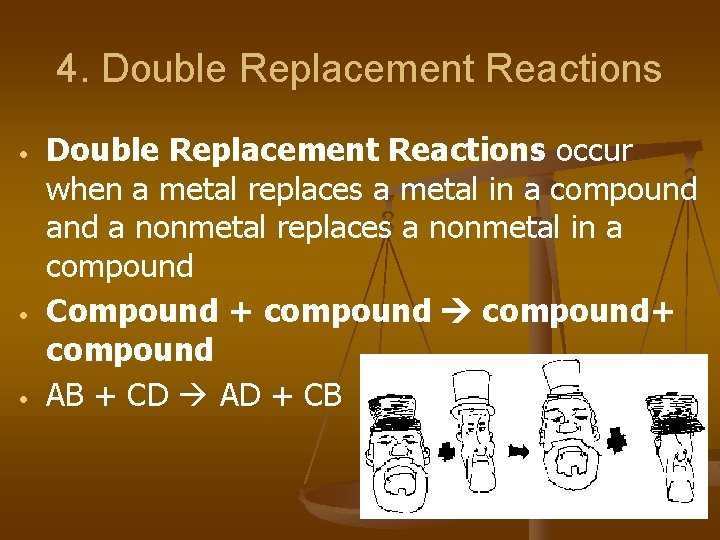4. Double Replacement Reactions • • • Double Replacement Reactions occur when a metal replaces a metal in a compound a nonmetal replaces a nonmetal in a compound Compound + compound+ compound AB + CD AD + CBDouble Replacement Reactions • • • Think about it like “foil”ing in algebra, first and last ions go together + inside ions go together Example: Ag. NO 3(aq) + Na. Cl(s) Ag. Cl(s) + Na. NO 3(aq) Another example: K 2 SO 4(aq) + Ba(NO 3)2(aq) 2 KNO 3(aq) + Ba. SO 4(s)Practice • Predict the products. Balance the equation 5. HCl(aq) + Ag. NO 3(aq) Ca. Cl 2(aq) + Na 3 PO 4(aq) Pb(NO 3)2(aq) + Ba. Cl 2(aq) Fe. Cl 3(aq) + Na. OH(aq) H 2 SO 4(aq) + Na. OH(aq) 6. KOH(aq) + Cu. SO 4(aq) 1. 2. 3. 4.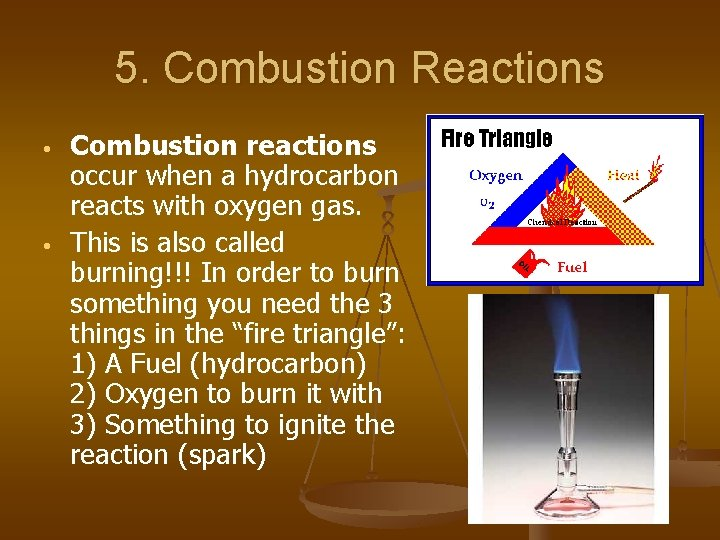5. Combustion Reactions • • Combustion reactions occur when a hydrocarbon reacts with oxygen gas. This is also called burning!!! In order to burn something you need the 3 things in the “fire triangle”: 1) A Fuel (hydrocarbon) 2) Oxygen to burn it with 3) Something to ignite the reaction (spark)Combustion Reactions • • • In general: Cx. Hy + O 2 CO 2 + H 2 O Products in combustion are ALWAYS carbon dioxide and water. (although incomplete burning does cause some byproducts like carbon monoxide) Combustion is used to heat homes and run automobiles (octane, as in gasoline, is C 8 H 18)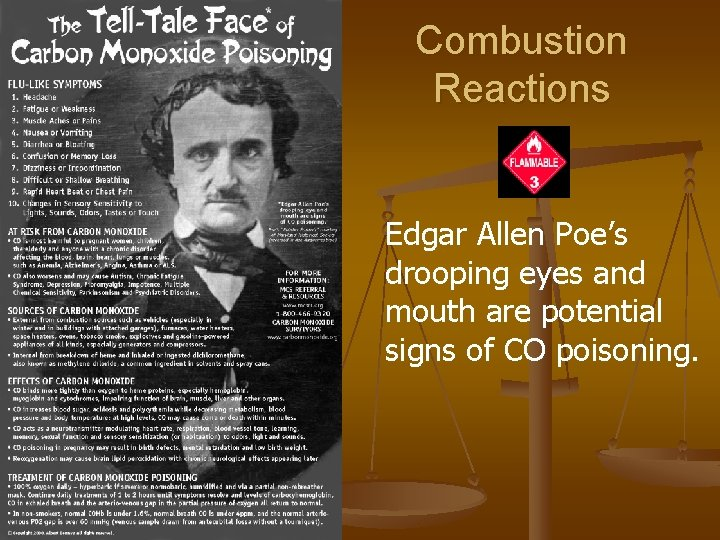Combustion Reactions Edgar Allen Poe’s drooping eyes and mouth are potential signs of CO poisoning.Combustion • Example • • C 5 H 12 + 8 O 2 5 CO 2 + 6 H 2 O Write the products and balance the following combustion reaction: • C 10 H 22 + O 2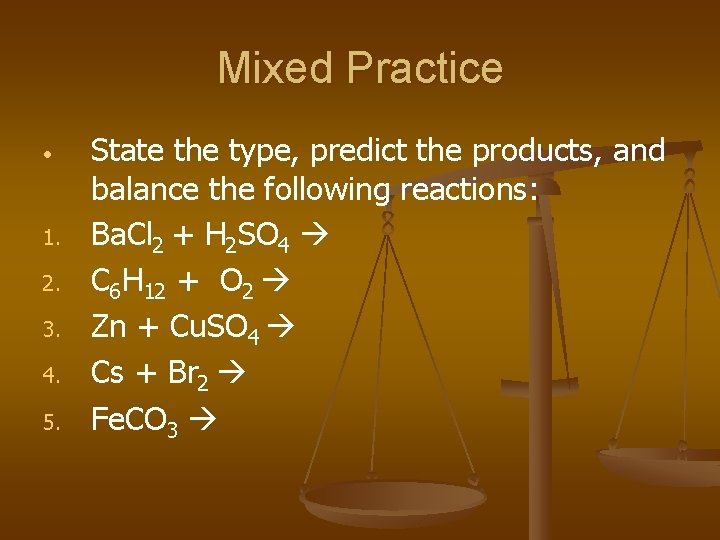Mixed Practice • 1. 2. 3. 4. 5. State the type, predict the products, and balance the following reactions: Ba. Cl 2 + H 2 SO 4 C 6 H 12 + O 2 Zn + Cu. SO 4 Cs + Br 2 Fe. CO 3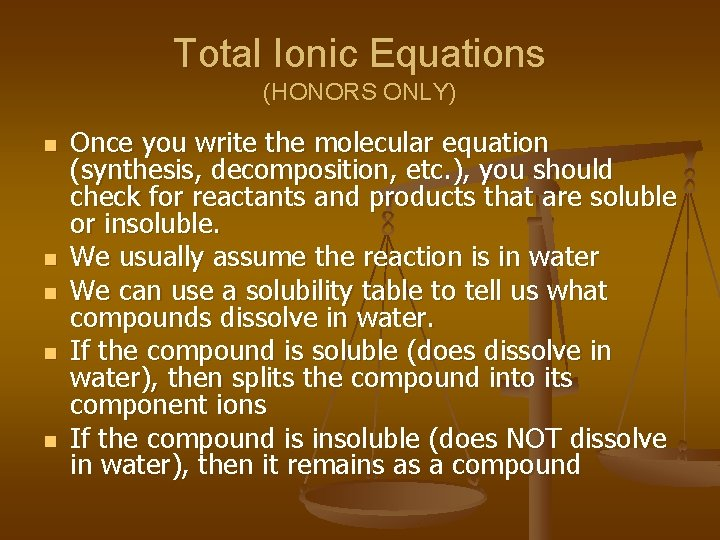Total Ionic Equations (HONORS ONLY) n n n Once you write the molecular equation (synthesis, decomposition, etc. ), you should check for reactants and products that are soluble or insoluble. We usually assume the reaction is in water We can use a solubility table to tell us what compounds dissolve in water. If the compound is soluble (does dissolve in water), then splits the compound into its component ions If the compound is insoluble (does NOT dissolve in water), then it remains as a compound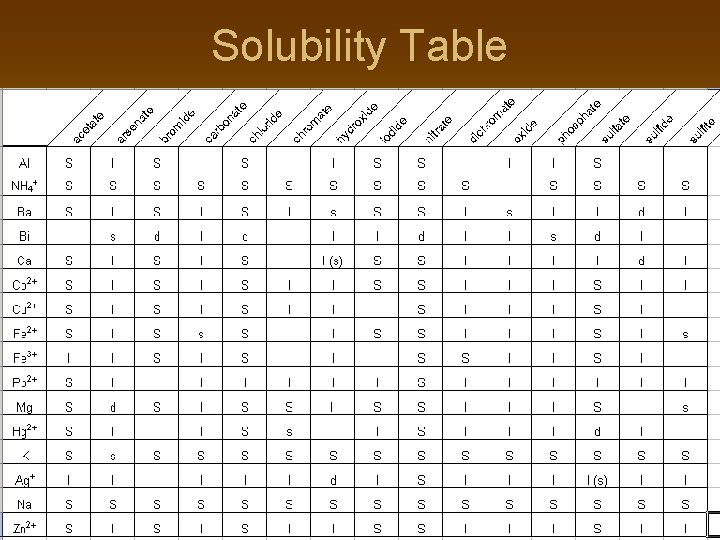Solubility Table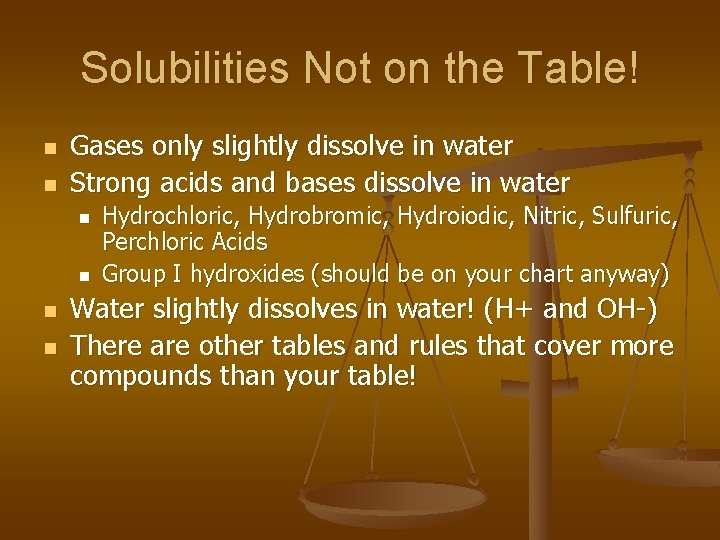Solubilities Not on the Table! n n Gases only slightly dissolve in water Strong acids and bases dissolve in water n n Hydrochloric, Hydrobromic, Hydroiodic, Nitric, Sulfuric, Perchloric Acids Group I hydroxides (should be on your chart anyway) Water slightly dissolves in water! (H+ and OH-) There are other tables and rules that cover more compounds than your table!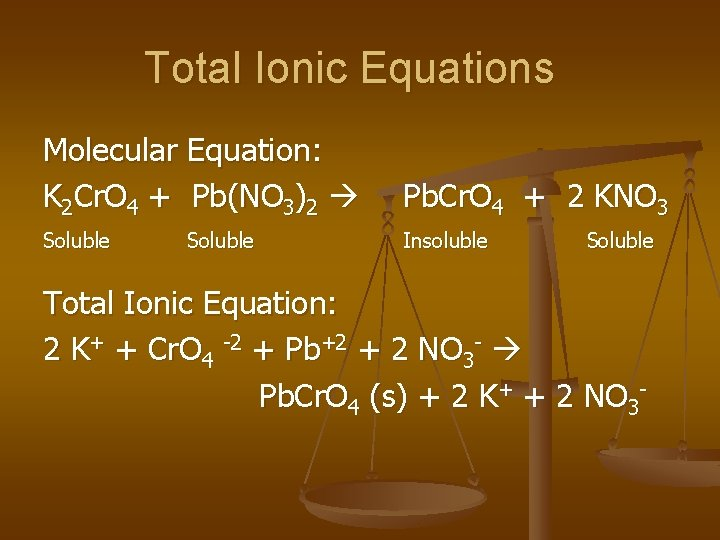Total Ionic Equations Molecular Equation: K 2 Cr. O 4 + Pb(NO 3)2 Pb. Cr. O 4 + 2 KNO 3 Soluble Insoluble Soluble Total Ionic Equation: 2 K+ + Cr. O 4 -2 + Pb+2 + 2 NO 3 - Pb. Cr. O 4 (s) + 2 K+ + 2 NO 3 -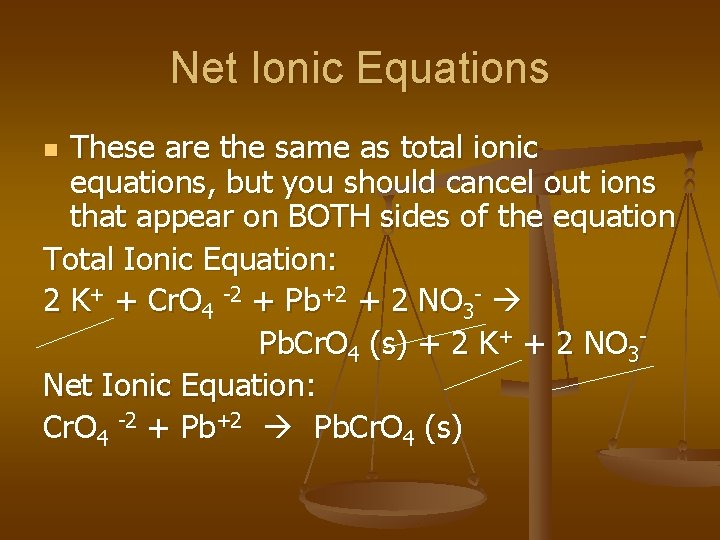Net Ionic Equations These are the same as total ionic equations, but you should cancel out ions that appear on BOTH sides of the equation Total Ionic Equation: 2 K+ + Cr. O 4 -2 + Pb+2 + 2 NO 3 - Pb. Cr. O 4 (s) + 2 K+ + 2 NO 3 Net Ionic Equation: Cr. O 4 -2 + Pb+2 Pb. Cr. O 4 (s) nNet Ionic Equations n Try this one! Write the molecular, total ionic, and net ionic equations for this reaction: Silver nitrate reacts with Lead (II) Chloride in hot water. Molecular: Total Ionic: Net Ionic: